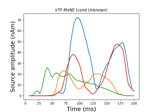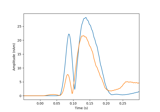# mne.inverse_sparse.tf_mixed_norm¶

mne.inverse_sparse.tf_mixed_norm(evoked, forward, noise_cov, loose='auto', depth=0.8, maxit=3000, tol=0.0001, weights=None, weights_min=0.0, pca=True, debias=True, wsize=64, tstep=4, window=0.02, return_residual=False, return_as_dipoles=False, alpha=None, l1_ratio=None, dgap_freq=10, rank=None, pick_ori=None, n_tfmxne_iter=1, verbose=None)[source]

Time-Frequency Mixed-norm estimate (TF-MxNE).

Compute L1/L2 + L1 mixed-norm solution on time-frequency dictionary. Works with evoked data  .

Parameters
evokedinstance of Evoked

Evoked data to invert.

forwarddict

Forward operator.

noise_covinstance of Covariance

Noise covariance to compute whitener.

loosefloat | ‘auto’ | dict

Value that weights the source variances of the dipole components that are parallel (tangential) to the cortical surface. Can be:

• float between 0 and 1 (inclusive)

If 0, then the solution is computed with fixed orientation. If 1, it corresponds to free orientations.

• 'auto' (default)

Uses 0.2 for surface source spaces (unless fixed is True) and 1.0 for other source spaces (volume or mixed).

• dict

Mapping from the key for a given source space type (surface, volume, discrete) to the loose value. Useful mostly for mixed source spaces.

depth

How to weight (or normalize) the forward using a depth prior. If float (default 0.8), it acts as the depth weighting exponent (exp) to use, which must be between 0 and 1. None is equivalent to 0, meaning no depth weighting is performed. It can also be a dict containing keyword arguments to pass to mne.forward.compute_depth_prior() (see docstring for details and defaults). This is effectively ignored when method='eLORETA'.

Changed in version 0.20: Depth bias ignored for method='eLORETA'.

maxitint

Maximum number of iterations.

tolfloat

Tolerance parameter.

weights

Weight for penalty in mixed_norm. Can be None or 1d array of length n_sources or a SourceEstimate e.g. obtained with wMNE or dSPM or fMRI.

weights_minfloat

Do not consider in the estimation sources for which weights is less than weights_min.

pcabool

If True the rank of the data is reduced to true dimension.

debiasbool

Remove coefficient amplitude bias due to L1 penalty.

wsize

Length of the STFT window in samples (must be a multiple of 4). If an array is passed, multiple TF dictionaries are used (each having its own wsize and tstep) and each entry of wsize must be a multiple of 4. See .

tstep

Step between successive windows in samples (must be a multiple of 2, a divider of wsize and smaller than wsize/2) (default: wsize/2). If an array is passed, multiple TF dictionaries are used (each having its own wsize and tstep), and each entry of tstep must be a multiple of 2 and divide the corresponding entry of wsize. See .

window

Length of time window used to take care of edge artifacts in seconds. It can be one float or float if the values are different for left and right window length.

return_residualbool

If True, the residual is returned as an Evoked instance.

return_as_dipolesbool

If True, the sources are returned as a list of Dipole instances.

alphafloat in [0, 100) or None

Overall regularization parameter. If alpha and l1_ratio are not None, alpha_space and alpha_time are overridden by alpha * alpha_max * (1. - l1_ratio) and alpha * alpha_max * l1_ratio. 0 means no regularization, 100 would give 0 active dipole.

l1_ratiofloat in [0, 1] or None

Proportion of temporal regularization. If l1_ratio and alpha are not None, alpha_space and alpha_time are overridden by alpha * alpha_max * (1. - l1_ratio) and alpha * alpha_max * l1_ratio. 0 means no time regularization a.k.a. MxNE.

dgap_freq

The duality gap is evaluated every dgap_freq iterations.

rankNone | dict | ‘info’ | ‘full’

This controls the rank computation that can be read from the measurement info or estimated from the data. See Notes of mne.compute_rank() for details.The default is None.

New in version 0.18.

pick_oriNone | “normal” | “vector”

Options:

• None

Pooling is performed by taking the norm of loose/free orientations. In case of a fixed source space no norm is computed leading to signed source activity.

• "normal"

Only the normal to the cortical surface is kept. This is only implemented when working with loose orientations.

• "vector"

No pooling of the orientations is done, and the vector result will be returned in the form of a mne.VectorSourceEstimate object.

n_tfmxne_iterint

Number of TF-MxNE iterations. If > 1, iterative reweighting is applied.

verbose

If not None, override default verbose level (see mne.verbose() and Logging documentation for more). If used, it should be passed as a keyword-argument only.

Returns
stcinstance of SourceEstimate

Source time courses.

residualinstance of Evoked

The residual a.k.a. data not explained by the sources. Only returned if return_residual is True.

References

1

A. Gramfort, D. Strohmeier, J. Haueisen, M. Hämäläinen, M. Kowalski “Time-Frequency Mixed-Norm Estimates: Sparse M/EEG imaging with non-stationary source activations”, Neuroimage, Volume 70, pp. 410-422, 15 April 2013. DOI: 10.1016/j.neuroimage.2012.12.051

2

A. Gramfort, D. Strohmeier, J. Haueisen, M. Hämäläinen, M. Kowalski “Functional Brain Imaging with M/EEG Using Structured Sparsity in Time-Frequency Dictionaries”, Proceedings Information Processing in Medical Imaging Lecture Notes in Computer Science, Volume 6801/2011, pp. 600-611, 2011. DOI: 10.1007/978-3-642-22092-0_49

3(1,2)

Y. Bekhti, D. Strohmeier, M. Jas, R. Badeau, A. Gramfort. “M/EEG source localization with multiscale time-frequency dictionaries”, 6th International Workshop on Pattern Recognition in Neuroimaging (PRNI), 2016. DOI: 10.1109/PRNI.2016.7552337

## Examples using mne.inverse_sparse.tf_mixed_norm¶